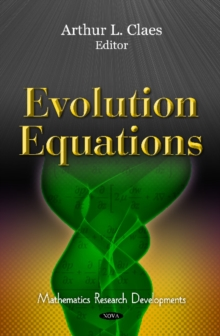Supporting your high street Find out how »
• My AccountBasket# Evolution Equations Hardback

## Edited by Arthur L. Claes

#### Description

This book presents topical research in the study of evolution equations.

Topics discussed include understanding the theory of noisy dynamical systems in Markovian and non-Markovian settings using evolution equations; Einstein's evolution equation for fluid with exponential free paths distribution; a theorem on the optical cubic-quintic Schrodinger equation; methods for solving non-linear partial differential equations based on Riccati equations; homogeneous and non-linear generalised master equations and the cosmological linear and non-linear growth in the accelerating Universe.

#### Information

• Format: Hardback
• Pages: 520 pages, Illustrations
• Publisher: Nova Science Publishers Inc
• Publication Date:
• Category: Mathematics
• ISBN: 9781612096520

£122.99

£99.35

on all orders

###### Pick up orders

from local bookshops